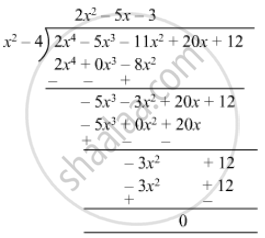# Obtain All the Zeroes of the Polynomial 2x4 − 5x3 − 11x2 + 20x + 12 When 2 and − 2 Are Two Zeroes of the Above Polynomial. - Mathematics

Sum

Obtain all the zeroes of the polynomial 2x4 − 5x3 − 11x+ 20x + 12 when 2 and − 2 are two zeroes of the above polynomial.

#### Solution

We know that if x = α is a zero of a polynomial, and then x - α is a factor of f(x).
Since 2 and −2 are zeros of f(x).
Therefore
(x − 2)(x + 2) = x2−4
(x2 − 4) is a factor of f(x).
Now, we divide 2x4 − 5x3 − 11x2 + 20x +12 by g(x) = (x2 − 4) to find the zero f(x).By using division algorithm we have f(x) = g(x) x q(x) - r(x)

2x4 − 5x3 − 11x2 + 20x + 12 = (x2 − 4)(2x2 − 5x − 3)
=(x − 2)(x + 2)[2x(x − 3) + 1(x − 3)]
=(x − 2)(x + 2)(x − 3)(2x + 1)

Hence, the zeros of the given polynomial are 2, −2, 3, −1/2.

Concept: Concept of Polynomials
Is there an error in this question or solution?